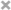# Xiuchuan Zhang

Personal Website

This is Xiuchuan's personal website.
I plan to post some of my current learning and review notes on it.
If you have any questions or suggestions, welcome to comment in my posts.

Coursera.org 机器学习 笔记
These are the notes by learning Andrew Ng’s “Machine Learning” from Coursera.org for future review

## Machine Learning

• Grew out of work in AI
• New capability for computers

Examples:

Database mining
Large datasets from growth of automation/ web
E.g., Web click data, medical records, biology, engineering

Applications cannot program by hand
E.g., Autonomous helicopter, handwriting recognition, most of Natural Language Processing (NLP), Computer Vision

Self-customizing programs
E.g., Amazon, Netflix product recommendations

Understanding human learning (brain, real AI)

### Introduction

• Definition
• Arthur Samuel (1959). Machine Learning: Field of study that gives computers the ability to learn without being explicitly programmed
• Tom Mitchell (1995) Well-posed Leaning Problem: A computer program is said to learn from experience E with respect to some task T and some performance measure P, if its performance on T, as measured by P, improves with experience E.

## Machine Learning Algorithms:

### Supervised learning

• Regression: Predict continuous valued output
• Classification: Discrete valued output (0 or 1 or others)

### Unsupervised learning

• allows us to approach problems with little or no idea what our results should look like.
• We can derive structure from data where we do not necessarily know the effect of the variables.
• We can derive this structure by clustering the data based on relationships among the variables in the data
• There is no feedback based on the prediction results

• E.g., Organize computing clusters, social network analysis, market segmentation, astronomical data analysis

### Model representation

• Notation
• m = Number of training examples
• x’s = “input” variable / features
• y’s = “output” variable / “target” variable
• Hypothesis
• Training set → Learning Algorithm → Hypothesis (h)
• xh → estimated value of y
• h: X → Y
• h maps x’s to y’s
• Linear regression with one variable or Univariate linear regression
• $h_{\theta}(x) = \theta_{0} + \theta_{1}x$
• shorthand: $h(x)$
• $\theta_{i}$’s: parameters

### Cost function

• Idea: Choose $\theta_{0}, \theta_{1}$ so that $h_{\theta}(x)$ is close to y for our training examples (x,y)
• $\underset{ \theta_{0}, \ \theta_{1} }{ \mathrm{minimize} }$ $\dfrac{1}{2m} \displaystyle\sum_{i=1}^m ( \hat{y}^{i}- y^{i} )^2 =$ $\dfrac{1}{2m} \displaystyle\sum _{i=1}^m (h_\theta (x^{i}) - y^{i} )^2$
• $h_{\theta}(x^{i}) = \theta_{0} + \theta_{1}x^{i}$
• Cost function: $J(\theta_0, \theta_1) =$ $\displaystyle \dfrac{1}{2m} \sum _{i=1}^m ( \hat{y}^{i}- y^{i} )^2 = \dfrac{1}{2m} \sum _{i=1}^m (h_\theta (x^{i}) - y^{i} )^2$
• Octave: J = 1/(2*m)*sum((X*theta .- y).^2)
• also called squared error cost function or squared error function
• Goal: $\displaystyle \underset{ \theta_{0}, \ \theta_{1} }{ \mathrm{minimize} } J(\theta_0, \theta_1)$

• contour plots or contour figures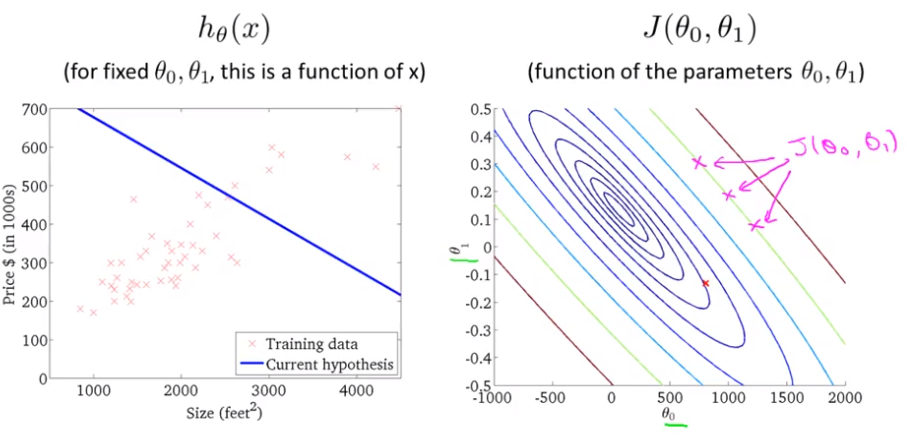• Outline:
• Start with some $\theta_{0}, \ \theta_{1}$
• Keep changing $\theta_{0}, \ \theta_{1}$ to reduce $J(\theta_{0}, \theta_{1})$ until we hopefully end up at a minimum
• Algorithm
• repeat until convergence { $\theta_j := \theta_j - \alpha \displaystyle \frac{\partial}{\partial \theta_j} J(\theta_0, \ \theta_1) \ \ (\mathrm{for} \ j = 0 \ \mathrm{and} \ j = 1 )$ }
• α: learning rate: too small → slow ; too large → overshoot; proper → automatically decrease α over time
• Correct simultaneous update
• temp0 := $\theta_0 - \alpha \displaystyle \frac{\partial}{\partial \theta_0} J(\theta_0, \ \theta_1) \ \ (\mathrm{for} \ j = 0 \ \mathrm{and} \ j = 1 )$
• temp1 := $\theta_1 - \alpha \displaystyle \frac{\partial}{\partial \theta_1} J(\theta_0, \ \theta_1) \ \ (\mathrm{for} \ j = 0 \ \mathrm{and} \ j = 1 )$
• $\theta_0 :=$ temp0
• $\theta_1 :=$ temp1
• linear regression:
• repeat until convergence:
• $\theta_0 := \theta_0 - \alpha \frac{1}{m} \sum\limits_{i=1}^{m}(h_\theta(x_{i}) - y_{i})$
• $\theta_1 := \theta_1 - \alpha \frac{1}{m} \sum\limits_{i=1}^{m}((h_\theta(x_{i}) - y_{i}) x_{i})$
• Octave: theta = theta - alpha/m*(X'*(X*theta .- y))
• Batch”: Each step of gradient descent uses all the training examples

## Multivariate Linear Regression

### Multiple Features (variables)

• n = number of features
• $x^{(i)} \text{ for } (i\in { 1,\dots, m } )$ = input (features) of $i^{th}$ training examples
• $x^{i} = [ x_{1}^{i} \ x_{2}^{i} \ \dots \ x_{n}^{i} ]^{T} \in \mathbb{R}^{n}$
• $x_{j}^{(i)}$ = value of $j^{th}$ features in $i^{th}$ training examples
• Hypothesis: $h_{\theta}(x^{i}) = \theta_{0} + \theta_{1}x_{1}^{i} + \theta_{2}x_{2}^{i} + \dots + \theta_{n}x_{n}^{i}$
• $x = [ x_{0} \ x_{1} \ x_{2} \ \dots \ x_{n} ]^{T} \in \mathbb{R}^{n+1} \ \ (x_{0}^{i} = 1)$
• $\theta = [ \theta_{0} \ \theta_{1} \ \theta_{2} \ \dots \ \theta_{n} ]^{T} \in \mathbb{R}^{n+1}$
• $h_{\theta}(x) = \theta_{0}x_{0} + \theta_{1}x_{1} + \theta_{2}x_{2} + \dots + \theta_{n}x_{n} \ \ (x_{0} = 1)$
$\ \ \ \ \ = \theta^{T}x$
• Parameters: $\theta_{0}, \theta_{1}, \dots , \theta_{n}$
• Cost function: $J(\theta) = J(\theta_{0}, \theta_{1}, \dots , \theta_{n}) =$ $\dfrac{1}{2m} \displaystyle\sum_{i=1}^m (h_\theta (x^{i}) - y^{i} )^2$
$\hspace{3em} J(\theta) = \dfrac{1}{2m} \displaystyle\sum_{i=1}^{m}((\sum_{j=0}^{n}\theta_j x^{(i)}_j) - y^{(i)})^2$
Repeat {
$\theta_j := \theta_j - \alpha \displaystyle \frac{\partial}{\partial \theta_j} J(\theta)$
} (simultaneously update for every $j = 0, \dots, n$ )
• New algorithm (n ≥ 1):
Repeat {
$\theta_j := \theta_j - \alpha \displaystyle \frac{1}{m} \sum\limits_{i=1}^{m}(h_\theta(x^{(i)}) - y^{(i)}) \cdot x_{j}^{(i)}$
} (simultaneously update $\theta_j$ for $j = 0, \dots, n$ )

• Features Scaling
• Let $x = \displaystyle \frac{x}{ \mathrm{range} S }$
to get every feature into approximately a $-1 \leq x \leq 1$ range
• Mean normalization: replace $x_j \mathrm{with} \frac{x_j - \mu_j}{ s_{j} }$ (Do not apply to $x_0 = 1$)
into $-0.5 \leq x_j \leq 0.5$ range
• Learning Rate choose
• “Debugging”: plot ( $\displaystyle\underset{ \theta }{ \mathrm{min} } \ J(\theta)$, No. of iterations)
$J(\theta)$ should decrease after every iteration → converge (sufficiently α)
• Not working → Use smaller α
slow converge → use larger α
• Create new feature
• Change the behavior or curve of our hypothesis function by making it a quadratic, cubic or square root function or any other form (Features Scaling)

### Normal equation

• Definition: Method to solve for θ analytically
• Formula: $\displaystyle\theta = (X^{T} X)^{-1} X^{T} y$
• Octave: pinv(x'*x)*x'*y
• No need feature scaling
• If n is very large, it works very slow, by compute $n^3$

## Classification

• Binary classification problem: y = 0 or 1
• Use linear regression:
• To map all predictions greater than 0.5 as a 1 and all less than 0.5 as a 0
• $h_\theta(x)$ can be >1 or <0, so it does not work well
• Logistic Regression: make $0 \le h_\theta(x) \le 1$

### Logistic Regression

• Clasification and Representation
• Sigmoid(Logistic) function: $g(Z) = \displaystyle\frac{1}{1+e^{-z}}$
• Octave: h = 1.0 ./ (1.0 + exp(-z))
• $h_\theta(x) = g(\theta^{T}x) = \displaystyle\frac{1}{1+e^{-\theta^{T}x}}$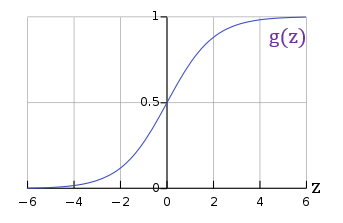• $h_\theta(x) =$ estimated probability that y = 1 on input x
• $h_\theta(x) = P(y=1 \vert x ; \theta) = 1 - P(y=0 \vert x ; \theta)$
• Decision boundary
• To get discrete 0 or 1 classification, can translate the output of the hypothesis function as:
• $h_\theta(x) \geq 0.5 \rightarrow y = 1$
• $h_\theta(x) \leq 0.5 \rightarrow y = 0$
• $g(z) \geq 0.5$ when $z \geq 0$ that $h_\theta(x) = g(\theta^T x) \geq 0.5$ when $\theta^T x \geq 0$
• From these statements we can say:
• $\theta^T x \geq 0 \Rightarrow y = 1$
• $\theta^T x \leq 0 \Rightarrow y = 0$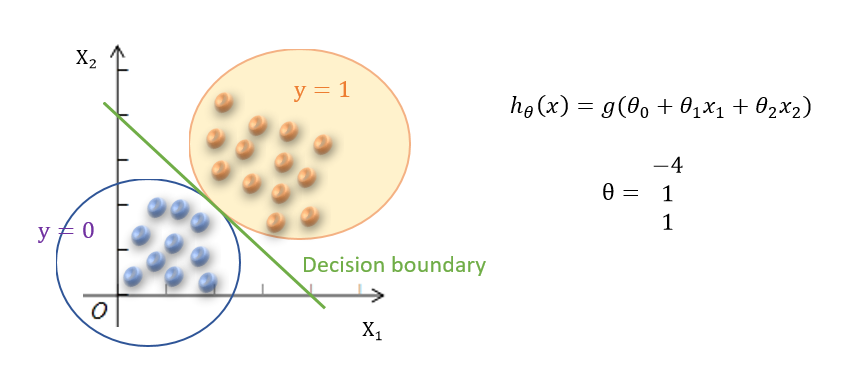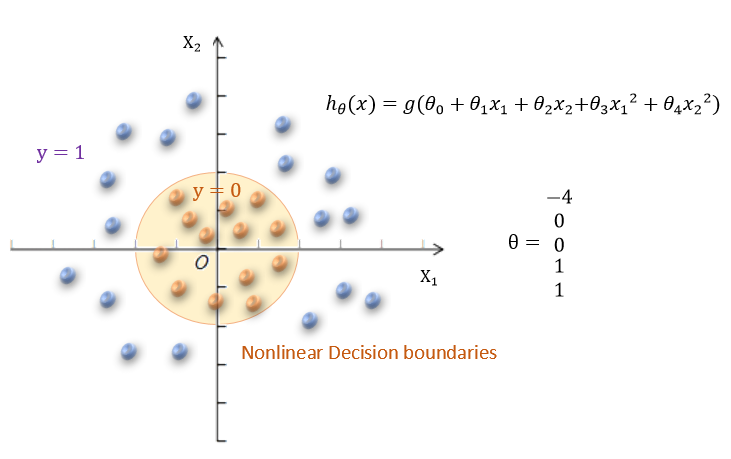• Cost function
• $J(\theta) = \displaystyle\dfrac{1}{m} \sum_{i=1}^m \mathrm{Cost}(h_\theta(x^{(i)}),y^{(i)})$
• $\mathrm{Cost}(h_\theta(x),y) = -\log(h_\theta(x))$ if y = 1
• $\mathrm{Cost}(h_\theta(x),y) = -\log(1-h_\theta(x))$ if y = 0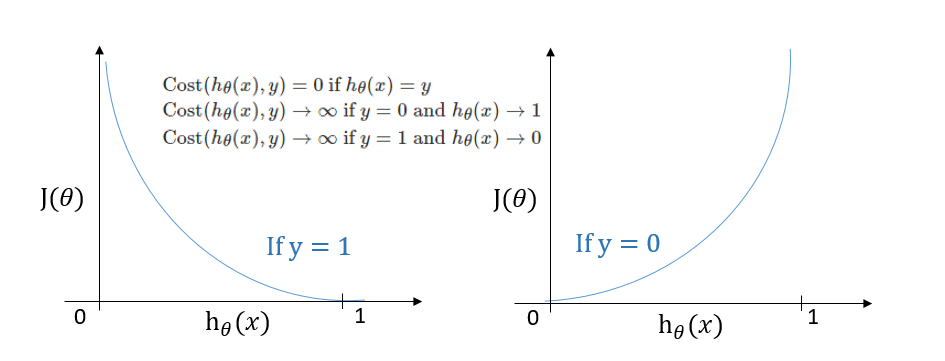• Compress: $\displaystyle \mathrm{Cost}(h_\theta(x),y) = -y\log(h_\theta(x)) -(1-y)\log(1-h_\theta(x))$
• Vectorized implementation:
$h = g(X\theta)$
$\displaystyle J(\theta) = \frac{1}{m} \cdot (-y^{T}\log(h)-(1-y)^{T}\log(1-h))$
Octave: the cost: J = 1/m * (-y' * log(h)-(1-y)'*log(1-h))
the partial derivatives: grad = 1/m * X'*(h-y)
• Repeat {
general form:
$\displaystyle \theta_j := \theta_j - \alpha \dfrac{\partial}{\partial \theta_j}J(\theta)$
derivative part using calculus:
$\displaystyle \theta_j := \theta_j - \frac{\alpha}{m} \sum_{i=1}^m (h_\theta(x^{(i)}) - y^{(i)}) x_j^{(i)}$
}
• Vectorized implementation:
$\displaystyle \theta := \theta - \frac{\alpha}{m} X^T (g(X\theta) - \vec y)$
• Optimization algorithm
• BFGS
• L-BFGS
• Codes in Octave:
function [jVal, gradient] = costFunction(theta)
jVal = [...code to compute J(theta)...];
gradient = [...code to compute derivative of J(theta)...]; end
• use octave’s ‘fminunc’ optimization algorithm
options = optimset('GradObj', 'on', 'MaxIter', 100);
initialTheta = zeros(2,1);
[optTheta, functionVal, exitFlag] = fminunc(@costFunction, initialTheta, options);
• Multiclass Classification: One-vs-all
• Train a logistic regression classifier $h_\theta^{(i)}(x)$ for each class i to predict the probability that $y = i$
$h_\theta^{(i)}(x) = P(y = i| x;\theta) (i=1,2,\dots,n)$
• On a new input x, to make a prediction, pick the class i that maximizes
prediction = $\displaystyle\underset{i}{\mathrm{max}} h_\theta^{(i)}(x)$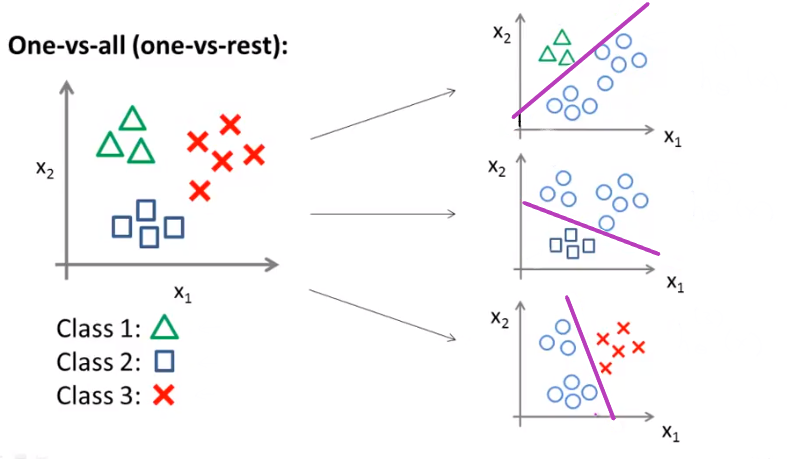## Overfitting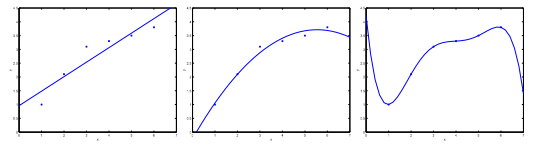• “Underfit” (“high bias”): not fit the training data very well
• “Just right”
• “Overfit” (“high variance”): may fit the trainning set very well, but fail to generalize to new examples

### Regularization

• Using the cost function with the extra summation, we can smooth the output of our hypothesis function to reduce overfitting.
$min_\theta\, \dfrac{1}{2m}\, \displaystyle[\sum_{i=1}^m (h_\theta(x^{(i)}) - y^{(i)})^2 + \lambda \sum_{j=1}^n \theta_j^2]$
• $\lambda$, or lambda is the regularization parameter
• If lambda is too large, it may cause underfitting.

### Linear Regression

Repeat {
$\displaystyle\theta_0 := \theta_0 - \alpha\, \frac{1}{m} \displaystyle\sum_{i=1}^m (h_\theta(x^{(i)}) - y^{(i)})x_0^{(i)}$
$\displaystyle\theta_j := \theta_j - \alpha\, [ ( \frac{1}{m}\, \sum_{i=1}^m (h_\theta(x^{(i)}) - y^{(i)})x_j^{(i)}) + \frac{\lambda}{m}\theta_j ]$ $\displaystyle\quad j \in \lbrace 1,2…n\rbrace$
$\displaystyle\Rrightarrow \theta_j := \theta_j (1- \alpha\,\frac{\lambda}{m}) - \alpha\, \frac{1}{m}\, \sum_{i=1}^m (h_\theta(x^{(i)}) - y^{(i)})x_j^{(i)})$
}

• Normal Equation:
$\displaystyle\theta = ( X^TX + \lambda \cdot L )^{-1} X^Ty$
$\text{where}\, \, L =$ $% $

### Logistic Regression

• Cost function:
• $J(\theta) = - \displaystyle\frac{1}{m} \sum_{i=1}^m [ y^{(i)}\, \log (h_\theta (x^{(i)})) + (1 - y^{(i)})\, \log (1 - h_\theta(x^{(i)}))] + \frac{\lambda}{2m}\sum_{j=1}^n \theta_j^2$
• $\sum_{j=1}^n \theta_j^2$ means to explicitly exclude the bias term $\theta_0$
• Octave:
• the cost:
J = 1/m * (-y' * log(h)-(1-y)'*log(1-h)) + (lambda/(2*m))*theta'*theta
• the partial derivatives:
grad = 1/m * X'*(h-y) + (lambda/m)*theta
• $\displaystyle h_\theta(x) = \frac{1}{1+e^{-\theta^{T}x}}$
Repeat {
$\displaystyle\theta_0 := \theta_0 - \alpha\, \frac{1}{m} \displaystyle\sum_{i=1}^m (h_\theta(x^{(i)}) - y^{(i)})x_0^{(i)}$
$\displaystyle\theta_j := \theta_j (1- \alpha\,\frac{\lambda}{m}) - \alpha\, \frac{1}{m}\, \sum_{i=1}^m (h_\theta(x^{(i)}) - y^{(i)})x_j^{(i)})$ $\displaystyle\quad j \in \lbrace 1,2…n\rbrace$
}

## Neural Networks

• Origins: Algorithms that try to mimic the brain

### Model representation(NN)

• Neuron model: Logistic unit
$\begin{bmatrix}x_0 \newline x_1 \newline x_2 \newline \end{bmatrix}\rightarrow\begin{bmatrix}\ \ \ \newline \end{bmatrix}\rightarrow h_\theta(x)$
• Sigmoid (logistic) activation function: $g(Z) = \displaystyle\frac{1}{1+e^{-z}}$
• Parameters $\theta$ also called “weights”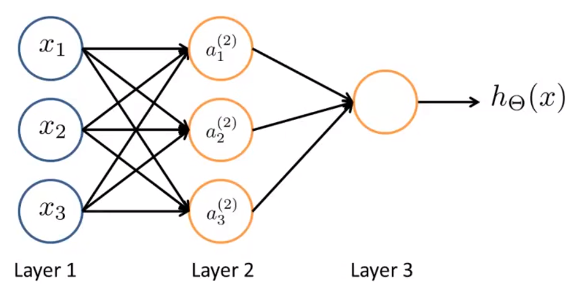• 1st layer called “input layer”; last layer called “output layer”; middle layers called “hidden layer”
• Notations
• $x_0 = 1$ is a “bias unit”
$\begin{bmatrix}x_0 \newline x_1 \newline x_2 \newline x_3\end{bmatrix}\rightarrow\begin{bmatrix}a_1^{(2)} \newline a_2^{(2)} \newline a_3^{(2)} \newline \end{bmatrix}\rightarrow h_\theta(x)$
• $a_i^{(j)} =$ “activation” of unit $i$ in layer $j$
• $\Theta^{(j)} =$ matrix of weights controlling function mapping from layer $j$ to layer $j+1$

\begin{align*} \displaystyle a_1^{(2)} = g(\Theta_{10}^{(1)}x_0 + \Theta_{11}^{(1)}x_1 + \Theta_{12}^{(1)}x_2 + \Theta_{13}^{(1)}x_3) \newline a_2^{(2)} = g(\Theta_{20}^{(1)}x_0 + \Theta_{21}^{(1)}x_1 + \Theta_{22}^{(1)}x_2 + \Theta_{23}^{(1)}x_3) \newline a_3^{(2)} = g(\Theta_{30}^{(1)}x_0 + \Theta_{31}^{(1)}x_1 + \Theta_{32}^{(1)}x_2 + \Theta_{33}^{(1)}x_3) \newline h_\Theta(x) = a_1^{(3)} = g(\Theta_{10}^{(2)}a_0^{(2)} + \Theta_{11}^{(2)}a_1^{(2)} + \Theta_{12}^{(2)}a_2^{(2)} + \Theta_{13}^{(2)}a_3^{(2)}) \newline \end{align*}

• If network has $s_j$ units in layer $\displaystyle j, s_{j+1}$ units in layer $j + 1$, then $\Theta^{(j)}$ will be of dimension $\displaystyle s_{j+1} \times (s_j + 1)$ that
$\Theta^{(j)} \in \displaystyle\Re^{s_{(j+1\ )}\ \times (s_j \ + 1)}$
• Forward propagation: Vectorized implementation
$\displaystyle z_k^{(2)} = \Theta_{k,0}^{(1)}\ x_0 + \Theta_{k,1}^{(1)}\ x_1 + \cdots + \Theta_{k,n}^{(1)}\ x_n$
%
• $a^{(1)} = x$
• $\displaystyle z^{(j)} = \Theta^{(j-1)} \ a^{(j-1)}$
\begin{align*}\displaystyle a_1^{(2)} = g(z_1^{(2)}) \newline a_2^{(2)} = g(z_2^{(2)}) \newline a_3^{(2)} = g(z_3^{(2)}) \newline \end{align*}
• $\hookrightarrow \displaystyle a^{(j)} = g(z^{(j)} )$
• Add $\displaystyle a_0^{(j)} = 1$
• $z^{(j+1)} = \Theta^{(j)} a^{(j)}$
• $\displaystyle h_\Theta(x) = \ a^{(j+1)} = \ g(z^{(j+1)}\ )$
• Other network architectures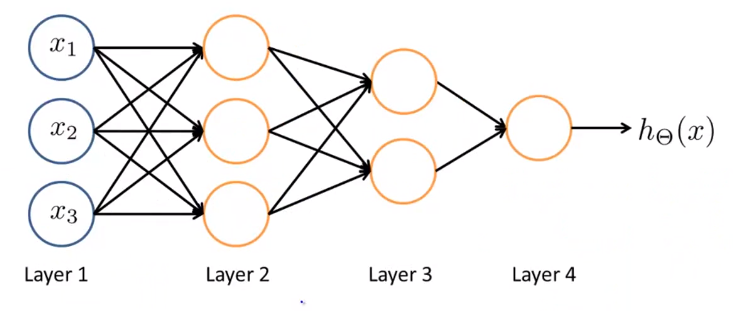## To be continued

• others: Reinforcement learning, recommender systems

• Practical advice for applying learning algorithms

Support